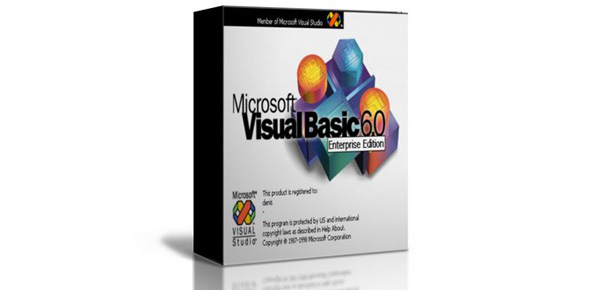# Visual Basic Ultimate Quiz: Trivia!

10 Questions | Total Attempts: 2303SettingsVisual Basic is an event-driven programming language made by Microsoft, released in 1991. It's easier to learn, write code and enables a programmer to create applications using various components. This quiz will test your knowledge about the functions, tools, and coding used in Visual Basic. Let's try it out.

• 1.
The following are versions of Visual Basic except:
• A.

Visual Basic 3.0

• B.

Visual Basic 3.0

• C.

Visual FoxPro

• D.

Visual Basic 3.0

• E.

Visual Basic 3.0

• 2.
Which of the following converts the expression to Byte data type in VB.NET?
• A.

CBool(expression)

• B.

CByte(expression)

• C.

CChar(expression)

• D.

CDate(expression)

• 3.
Which event is activated when a RadioButton is selected?
• A.

Checked

• B.

CheckedChanged

• C.

Selected

• D.

SelectedChanged

• 4.
Which is a type of procedure found in VB.Net?
• A.

Event

• B.

Function

• C.

Sub

• D.

All of the above.

• 5.
Which of the following statement declares the operator symbol, operands, and code that define an operator procedure on a class or structure?
• A.

Sub

• B.

Declare

• C.

Operator

• D.

Property

• 6.
Which of the following Collection class of VB.NET represents a last-in, first-out collection of the object?
• A.

ArrayList

• B.

Hashtable

• C.

SortedList

• D.

Stack

• 7.
The methodology where the code is broken into small, logical procedures is called:
• A.

Event-driven programming.

• B.

Functional programming.

• C.

Granular programming.

• D.

Modular programming.

• 8.
Which is an optional element of an event procedure?
• A.

End Sub

• B.

Handles

• C.

Statements

• D.

Sub

• 9.
Which of the following block of VB.NET identifies a block of code for which particular exceptions will be activated?
• A.

Try

• B.

Catch

• C.

Finally

• D.

Throw

• 10.
What happens when a parameter in a procedure is declared ByVal?
• A.

Only arguments of numeric data types are allowed.

• B.

A reference to the argument is sent to the procedure.

• C.

A copy of the argument is sent to the procedure.

• D.

All of the above.

Related TopicsBack to top